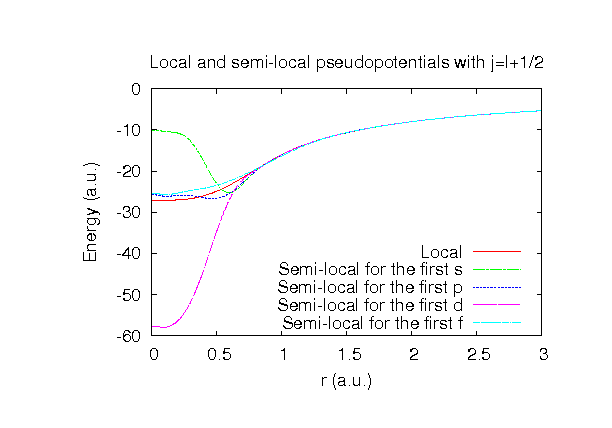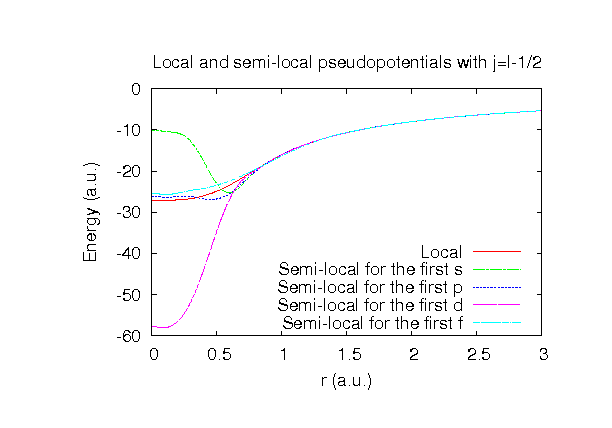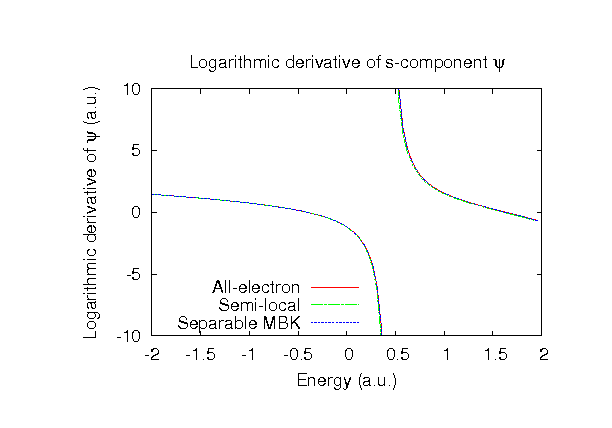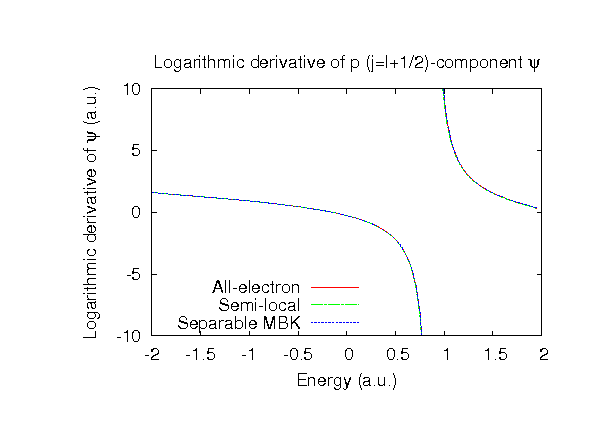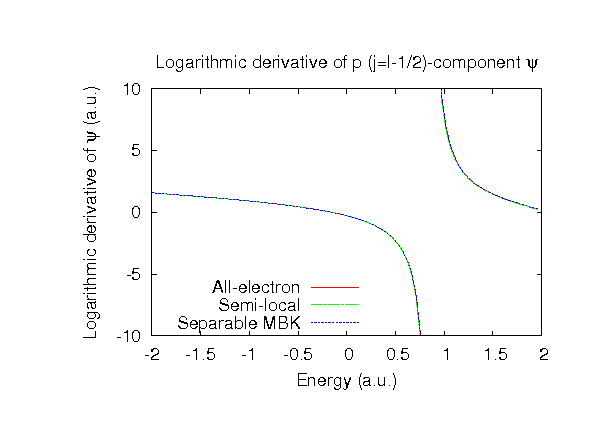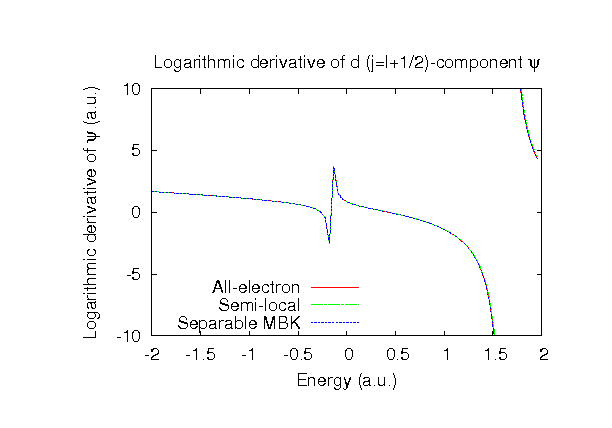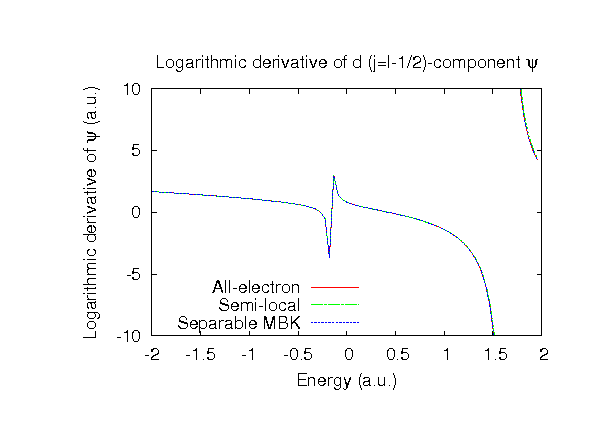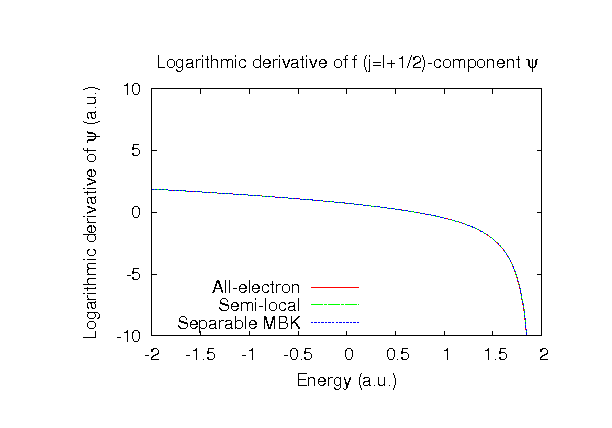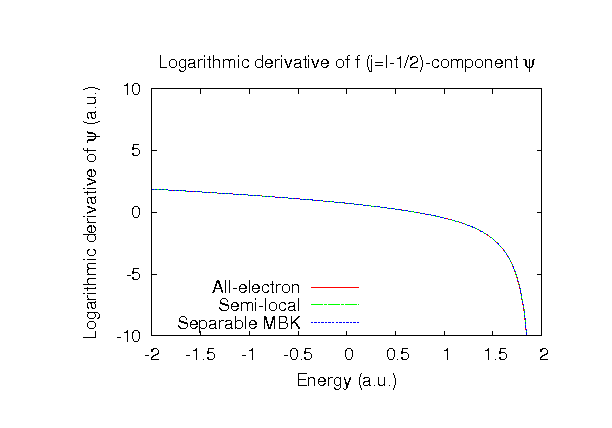### Fully relativistic pseudopotentials

Fully relativistic pseudopotentials generated by the MBK (PRB 47, 6728 (1993)) scheme within LDA (CA13) and GGA (PBE13) which contain a partial core correction and fully relativistic effects including spin-orbit coupling.

### Pseudo-atomic orbitals

The number below the symbol means a cutoff radius (a.u.) of the confinement potential. These file includes fifteen radial parts for each angular momentum quantum number l (=0,1,2,3). The basis functions were generated by variationally optimizing the corresponding primitive basis functions in the single atom and the BCC bulk. The input files used for the orbital optimization can be found at Fe_opt.dat and Febcc_opt.dat. Since Fe_CA13H.vps and Fe_PBE13H.vps include the 3s, 3p, 3d, and 4s states (16 electrons) as the valence states, the minimal basis set is Fe*.*H-s2p1d1. Our recommendation for the choice of cutoff radius of basis functions is that Fe6.0H.pao is enough for bulks, but Fe8.0H.pao or Fe10.0H.pao is preferable for molecular systems.

### Benchmark calculations by the PBE13 pseudopotential with the various basis functions

(1) Calculation of the total energy as a function of lattice constant in the bcc structure, where the total energy is plotted relative to the minimum energy for each case. a0 and B0 are the equilibrium lattice constant and bulk modulus obtained by fitting to the Murnaghan equation of state. The difference between Fe6.0H-s3p3d2 and Fe6.0H-s3p3d3f1 in the total energy at the minimum point is 0.106 eV/atom. An input file used for the OpenMX calculations can be found at Febcc-EvsV.dat. For comparison the result by the Wien2k code is also shown, where the calculation was performed by default setting in the Ver. 10.1 of Wien2k except for the use of RMT x KMAX of 14.(2) Calculations of the band dispersion in the bcc structure, where the spin polarized collinear calculations with the lattice constant of 2.87 Ang. were performed using Fe_PBE13H.vps and Fe6.0H-s3p3d2, and the origin of the energy is taken to be the Fermi level. The input file used for the OpenMX calculations can be found at Febcc-Band.dat. The magnetic moment per atom is also shown in the figures. For comparison the result by the Wien2k code is also shown, where the calculation was performed by default setting in the Ver. 10.1 of Wien2k except for the use of RMT x KMAX of 14.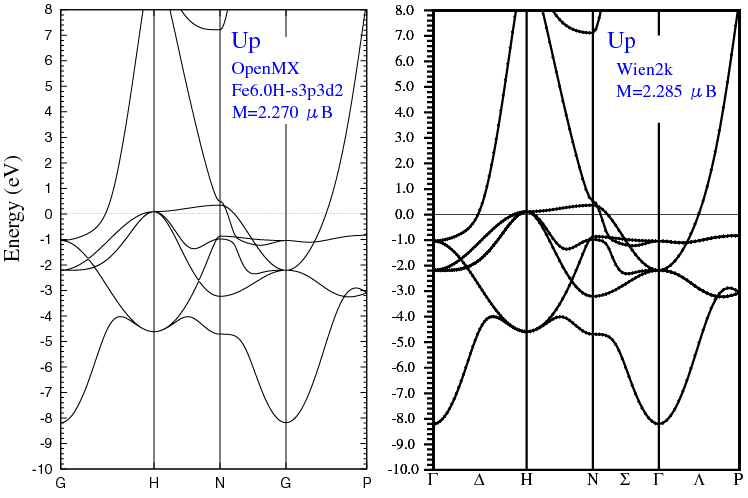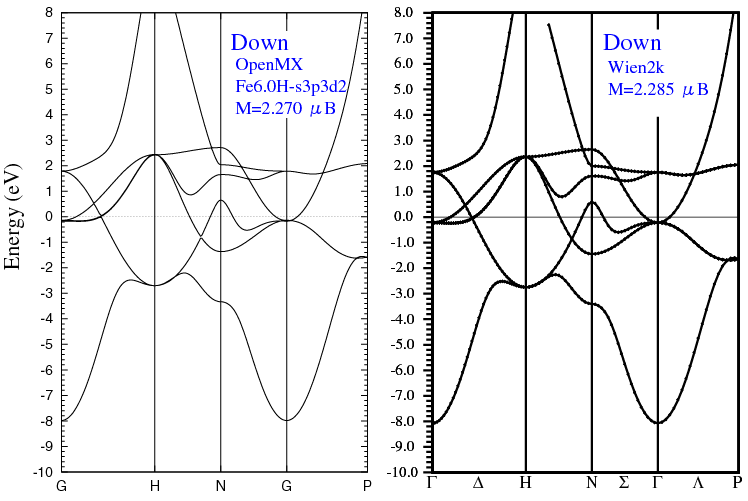(3) Calculations of vacancy formation energy, solution energy of Si atom, and vacancy-solute (Si atom) interaction energies in bcc-iron. The input files used for the OpenMX calculations can be found at Fe54.dat, Fe53v1.dat, Fe53Si1.dat, Fe52Si1FV1.dat, Fe52Si1SV1.dat, and Si8.dat. For all the calculations, basis sets of Si7.0-s3p3d2 and Fe-s3p3d2 were assigned for Si atom and vacancy, respectively, where the same cutoff radius as the basis set of Fe atom in the calculation was used for the basis set for the vacancy. The internal coordinates were fully optimized until the maximum force becomes less than 0.0001 Hartree/Bohr, while the lattice parameters were fixed during the optimization.

 Vacancy formation energya (eV) Solution energy of Si atomb (eV) Nearest neighbouring vacancy-solute interaction energyc (eV) Second nearest neighbouring vacancy-solute interaction energyd (eV) Fe5.0H-s2p2d1 2.38 -1.54 -0.282 -0.097 Fe5.0H-s2p2d2 2.44 -1.28 -0.338 -0.121 Fe5.5H-s2p2d1 2.28 -1.19 -0.315 -0.100 Fe5.5H-s2p2d2 2.36 -1.16 -0.321 -0.101 Fe6.0H-s2p2d1 2.09 -1.20 -0.316 -0.121 Fe6.0H-s2p2d2 2.23 -1.20 -0.309 -0.141 VASPe 2.21 -1.18 -0.286 -0.107

a The energy is defined as 54/53 EFe53 - EFe54, where EFe54 and EFe53 are the total energies of a supercell containing of 54 Fe atoms in the bcc structure and the supercell with a vacancy, respectively.
b The energy is defined as EFe53Si - 1/8 ESi8 - 53/54 EFe54, where EFe53Si and ESi8 are the total energies of the supercell containing 53 Fe atoms and a Si substitution atom, and a supercell containing 8 Si atoms in the diamond structure, respectively.
c The energy is defined as EFe52Si + EFe54 - EFe53 - EFe53Si, where EFe52Si is the total energy of the supercell containing 52 Fe atoms, a Si substitution atom, and a vacancy. The Si atom is situated to the vacancy as the nearest neighbour.
d The energy is defined as EFe52Si + EFe54 - EFe53 - EFe53Si, where EFe52Si is the total energy of the supercell containing 52 Fe atoms, a Si substitution atom, and a vacancy. The Si atom is situated to the vacancy as the second nearest neighbour.
e The corresponding VASP data were provided from Dr. Wakeda of Osaka University. The calculation condition is as follows: The PAW method and GGA-PBE were used. The cutoff energy for wave functions and charge density are about 334 and 511 eV, respectively. The occupation was treated by the Methfessel-Paxton method with a smearing of 0.2 eV.

### Supplementary information for the GGA (PBE13) pseudopotential